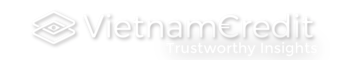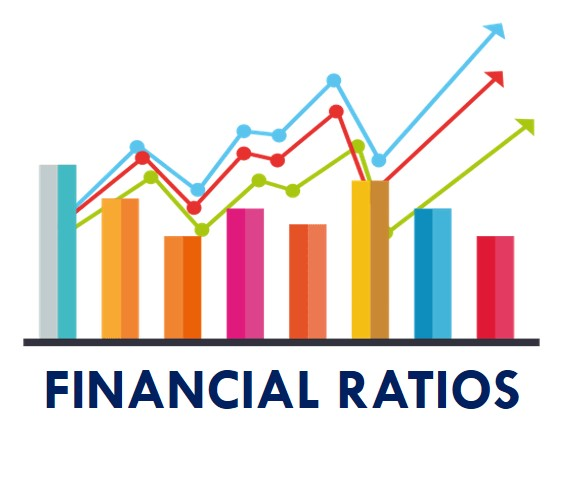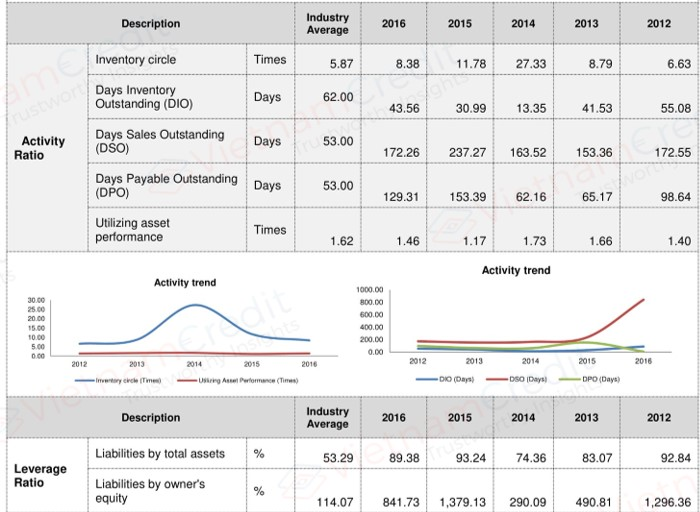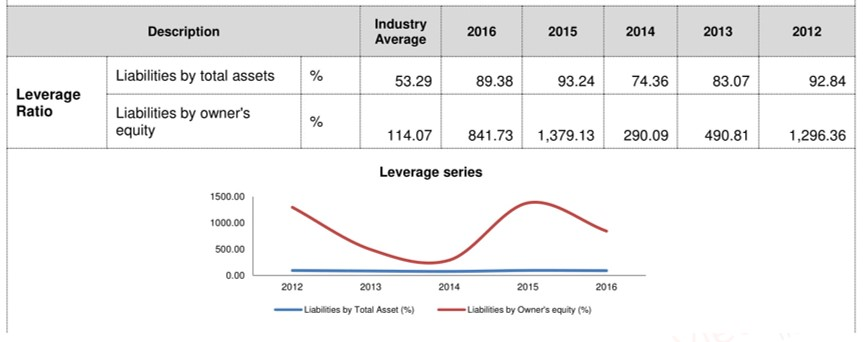0
USD
Go to cart# COMPANY FINANCIAL RATIOS: THE KEY TO STRENGTHEN YOUR DECISION! (Part 2)

Thursday 23, 04 2020
Every ratio provides its best applicability, yet it depends on your purpose and the reliability of a company’s data. This article will draw you several basic ideas of this topic, along with how to use it wisely.
2. Activity Ratios

Activity ratios are a class of financial metrics used to measure a firm's ability to convert different accounts within its balance sheets into cash or sales. Normally, people use them to measure the relative efficiency of a firm or determine whether a company's management is doing good enough. There are 4 commonly used activity ratios:- Days Inventory Outstanding (DIO)

Days inventory outstanding ratio, explained as an indicator of inventory turns is an important financial ratio for any company with inventory. It shows how quickly management can turn inventories into cash. In general, a decrease in DIO is an improvement to working capital, and an increase is a deterioration. The DIO is calculated as:

DIO = (average inventory / cost of goods sold) * 365 days

- Days Sales Outstanding

Days sales outstanding (DSO) is a measure of the average number of days that it takes a company to collect payment after a sale has been made. DSO is often determined on a monthly, quarterly or annual basis, and can be calculated by dividing the amount of accounts receivable during a given period by the total value of credit sales during the same period, and multiplying the result by the number of days in the period measured. The DSO is calculated as:

DSO = (accounts receivable / total credit sales) * 365 days

- Days Payable Outstanding

Days payable outstanding (DPO) is a company's average payable period that measures how long it takes a company to pay its invoices from trade creditors, such as suppliers. The DPO is calculated as:

DPO = (Ending account payable/cost of sale) * 365 days

Days payable outstanding is also referred to as the number of days of payables.

- Utilizing asset performance

Utilizing asset performance refers to a business' ability to take operational resources, manage them and produce profitable returns. It is the ability of a business to coax a positive performance out of its assets resulting in positive company performance. It measures how quickly operations in the company lead to revenue and how efficiently operations are being run.

Utilizing asset performance is typically used to compare one company's performance over time or against its competition. Possessing strong asset performance is one of the criteria for determining whether a company is considered a good investment. The utilizing asset performance is calculated as:

Utilizing asset performance = (net revenues / total assets) * 365 days

## 3. Leverage Ratios

Leverage ratios are a class of financial metrics that look at how much capital comes in the form of debt (loans) or assess the ability of a company to meet its financial obligations. Normally, people use them to finance a company operation evaluate whether it can pay its debts off as they come due. There are 4 commonly used leverage ratios:### - Liabilities by total assets ratio

The liabilities by total assets ratio is a leverage ratio that defines the total amount of debt relative to assets. This metric enables comparisons of leverage to be made across different companies. The higher the ratio, the higher the degree of leverage and, consequently, financial risk. The liabilities by total assets ratio is a broad ratio that includes long-term and short-term debt (borrowings maturing within one year), as well as all assets – tangible and intangible. It is calculated as:

Liabilities by total assets = Total liabilities / Total assets

### - Liabilities by capital ratio

The liabilities by capital ratio is a measurement of a company's financial leverage. It is calculated by taking the company's interest-bearing debt, both short- and long-term liabilities and dividing it by the total capital. Total capital is all interest-bearing debt plus shareholders' equity, which may include items such as common stock, preferred stock, and minority interest. The liabilities by capital ratio is calculated as:

Liabilities by capital ratio = Total liabilities / Total capital

### - Liabilities by owner's equity ratio

Liabilities by owner's equity ratio, calculated by dividing a company’s total liabilities by its stockholders' equity, is a debt ratio used to measure a company's financial leverage. Liabilities by owner's equity ratio indicates how much debt a company is using to finance its assets relative to the value of shareholders’ equity. The liabilities by owner's equity ratio is calculated as:

Liabilities by owner's equity = Total Liabilities / Shareholders' Equity

The result can be expressed either as a number or as a percentage. It is also referred to as a risk or gearing ratio.

### - Interest coverage ratio

The interest coverage ratio is a leverage ratio and profitability ratio used to determine how easily a company can pay interest on its outstanding debt. The interest coverage ratio may be calculated by dividing a company's earnings before interest and taxes (EBIT) during a given period by the company's interest payments due within the same period. The interest coverage ratio is calculated as:

Interest coverage ratio = EBIT / Interest payments

Where EBIT (Earnings before Interest and Taxes) = Net Income + Interest + Taxes

## 4. Profitability ratios

Profitability ratios are a class of financial metrics that are used to assess a business's ability to generate earnings relative to its associated expenses. Normally, people use them to indicate that the company is doing well or not. There are 4 commonly used profitability ratios:### - Return on assets

Return on assets (ROA) is an indicator of how profitable a company is relative to its total assets. ROA gives a manager, investor, or analyst an idea as to how efficient a company's management is at using its assets to generate earnings. Return on assets is displayed as a percentage and is calculated as:
ROA = EBIT / Total assets

### - Return on equity

Return on equity (ROE) is the amount of net income returned as a percentage of shareholders' equity. Return on equity (also known as "return on net worth" [RONW]) measures a corporation's profitability by revealing how much profit a company generates with the money shareholders have invested. ROE is expressed as a percentage and calculated as:

ROE = EBIT / Owner's equity

### - Gross profit margin

Gross profit margin is a financial metric used to assess a company's financial health and business model by revealing the proportion of money left over from revenues after accounting for the cost of goods sold. One can calculate gross profit margin, also known as gross margin, by dividing gross profit by revenues. It is calculated as:

GPM = Gross profit / Total revenue

### - Net profit margin

Net profit margin, or net margin, is equal to net income or profits divided by total revenue and represents how much profit each dollar of sales generates. Net profit margin is the ratio of net profits or net income to revenues for a company, business segment or product. Net profit margin is typically expressed as a percentage but can also be represented in decimal form. The net profit margin illustrates how much of each dollar collected by a company as revenue translates into profit. It is calculated as:

NPM = EBIT / Total revenue

>> COMPANY FINANCIAL RATIOS: THE KEY TO STRENGTHEN YOUR DECISION! (Part 1)
Categories

## You may also likeMonday 29, 06 2020

#### Fintech or Techfin: what would be the trend of banking industry?

Banking sector has been going through the overwhelming consecutive changes....Thursday 23, 04 2020

#### COMPANY FINANCIAL RATIOS: THE KEY TO STRENGTHEN YOUR DECISION! (Part 1)

Have you ever wondered “why economists invented so many financial ratios?”....
2
0